Email   Home Page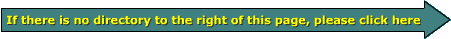Operational Amplifiers

Operational amplifiers (op amps) were originally used for mathematical operations in 'analog' computers. They typically have 2 inputs, a positive (non_inverting) input and a negative (inverting) input. A signal fed into the positive (non_inverting) input will produce an output signal which is in phase with the input. If the signal is fed into the negative (inverting) input, the output will be 180 degrees out of phase when compared to the input.

There are a bazillion (technical term) applications for op amps. The following section is an attempt to give you a basic understanding of just a few applications. None of the power supply connections are shown. Most op amp circuits used in audio use a ±15 volt power supply (especially when the audio equipment has a switching power supply). They can also be used with a single ended supply (no negative voltage) in head units and other such equipment that have no switching power supply.

The diagram below shows the schematic symbol for an op amp.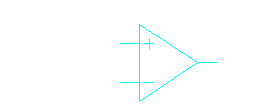Op-Amp Operation:
The circuit below shows a simple buffer circuit. The input impedance of an op amp is extremely high (on the order of 1012 ohms). It might be used if the input signal to the op amp was coming from a source which could supply almost no current. The output of the op amp can easily drive 1000 ohms or more. The output, when used as a buffer, will theoretically be identical to the input signal. I can't say it is identical because there is a small amount of distortion in all amplifier circuits. The distortion in this circuit would be EXTREMELY low and would most likely be inaudible.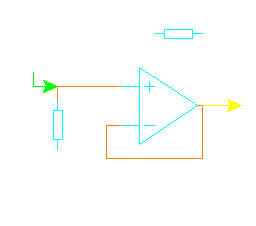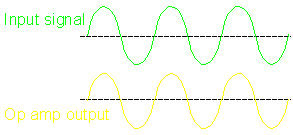Op-Amps as Amplifiers:
An op amp can also easily amplify a signal such as audio. The diagram below shows the circuit for an op amp that would give an output signal twice as large as the input. Op amps don't like errors. To get amplification, you induce an error in the signal going back to the negative input of the op amp. An op amp will do everything in it's power to get the signal on the negative input to match the signal on its positive input. To get an output that's twice as large as the input, you use 2 equal value resistors as a voltage divider to reduce the return (feedback) signal at the negative input by half. If the return signal doesn't match the input signal, the op amp will increase the output until the signal returned to the negative input is the same as the input to the positive input. Since the voltage divider cuts the signal in half, the signal at the output must be doubled. You can create any amount of gain needed by changing the value of ONE of the resistors in the 'feedback' path. The actual limit of gain will be determined by the op amp design. When using an op amp as a non-inverting amplifier, the gain will always be greater than or equal to 1. To get a gain of less than 1, you need to use a voltage divider on the input signal.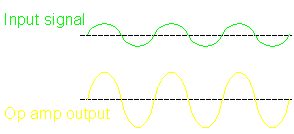Calculating Voltage Gain (non-inverting input):
By knowing the value of the feedback, inverting input resistor and input voltage, we can calculate the output voltage. The formula is:

Vout = Vin*((Rf/Ri)+1)

For equal value resistors of 4700 ohms and an input voltage of 4 volts...

Vout = 4*((4700/4700)+1)
Vout = 8

 Input Voltage? Feedback Resistor? Inv Input Resistor? Output Voltage:

Op-Amp Inverters:
As I said earlier, an op amp can produce a signal which is 180 degrees out of phase (inverted) with respect to the input signal. The diagram below shows an op amp used as an inverter. To use an op amp as an inverting amplifier, you must send the signal into the negative input instead of the positive input. As I said before, the op amp will do everything it possibly can to make the voltage (signal) on the the negative input match the positive input. In the following diagram, you can see that the positive input is connected to ground. It's shown as being connected through a resistor but the resistance to ground in unimportant. What is important is that the positive input has no signal (it's connected to the reference, ground). This means that the op amp's negative input will have no visible (voltage) signal on it. When you're driving the negative input it will act as a virtual ground. The input is converted from a voltage drive to a current drive. The change in current is what drives the op amp. This is important to know because when you look at the negative input with an oscilloscope, you will see no signal (when the circuit is an inverting amplifier). I said earlier that the op amp inputs had a very high impedance. While this is true, when using the inverting input with feedback (which is necessary for audio reproduction), the input impedance becomes the value of the input resistor.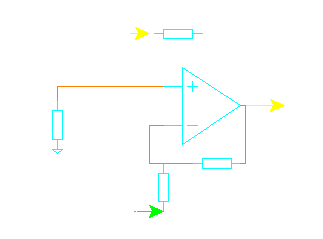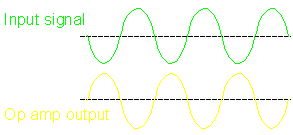Calculating Voltage Gain (inverting input):
By knowing the value of the feedback, inverting input resistor and input voltage, we can calculate the output voltage. The formula is:

Vout = Vin*(Rf/Ri)*-1

For equal value resistors of 2200 ohms and an input voltage of 3.5 volts...

Vout = 3.5*(2200/2200)*-1
Vout = -3.5

 Input Voltage? Feedback Resistor? Inv Input Resistor? Output Voltage:

Op-Amp Error Correction:
An op amp is commonly used in a circuit where error correction is required. Op amps can't (generally) supply a large amount of current at its output. If a signal is fed to the positive input of an op amp and the op amp is driving a circuit which CAN supply a large amount of current (like the regulator that we will work with later on this page), the output of the whole system can be fed back into the negative input of the op amp. This will allow the op amp to compare the output (of the whole system) to the input signal and correct as needed. If the op amp is used in a circuit which needs little current at its output, the op amp can still monitor the output and correct as needed.

If you read the servo page before this page, you'll remember that there was a reference point indicated by a green arrow. The positive input on the op amp is analogous to the green arrow. The height of the green arrow would be analogous to the voltage on the positive input. The sensor would be analogous to the valves which would also be analogous to the negative input of the op amp. The error correction would come at the output of the op amp (instead of the hydraulic actuator).

The next diagram has a resistor in series with the output of the op amp and the load which is to be driven by the op amp. The resistor represents anything that may be between the op amp and the load. The resistor could actually be a long run of wire, resistance in the copper of a printed circuit board or anything else that may cause the signal to be distorted. If the op amp didn't monitor the signal at the load, the signal would be distorted (in this case, the simple series resistance would only reduce the signal level). If the resistor would instead be an external circuit designed to increase the output current (such as the transistors, resistors, capacitors... of a power amplifier), the output of the op amp may not even resemble the final output signal. The op amp would do everything possible to get the final output to match the input signal. A look back at the amplifier page shows a circuit where an op amp is used for error correction in a power amplifier circuit.This calculator shows you how a servo circuit compensates for such things as wiring or anything else in-between the amplifier and the load. The output resistance simulates any series resistance or anything else that may distort the signal. The op amp tries to make the output voltage match the input voltage. As you take changes in the top 3 fields, watch how the output of the opamp changes to keep the input and output voltages equal. If you make the output resistance 0 ohms, you'll see that the opamp voltage and output voltage are equal because there is no output resistance to cause a voltage drop (distortion).

 Data Input: Input Voltage? Volts Output Resistance? Ohms Load Resistor? Ohms Data Output: Op-amp Output Voltage = Volts Output Voltage = Volts Output Current = Amps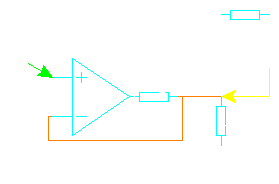Slightly More In-Depth Information

Op Amps as Comparators:
An op amp can be used to compare 2 different voltages. If you apply a reference voltage to one of the inputs and then use the other input to monitor a voltage from some point in a circuit, the output of the op amp will go from high to low (or vice-versa) as the monitored voltage crosses the reference voltage.

As you can see in the following demo, The yellow line shows the voltage on the negative input. The voltage follows a curve because the capacitor is charging. The capacitor charges faster at first then it slows as it approaches full charge. The blue line indicates the voltage on the positive input. Since the 2 voltage divider resistors are of equal value, the voltage on the positive input is exactly half of the power supply voltage. You can also see that the voltage on the output of the op amp is high (close to the power supply voltage). If you push the button, the capacitor will start to charge. The little lines in the window are voltage indicators. Think of them as a portion of the trace on an oscilloscope. As the capacitor charges (and the voltage starts to rise), the line goes up (it follows the voltage). As you can see, the voltage on the output does not change until the voltage on the negative input is higher than the voltage on the positive input. Remember that the previous circuits had a feedback signal return path between the output of the op amp to the negative input. Since there is no feedback, the gain is essentially (ideally) infinite. This will make the output swing from its maximum positive output voltage to its maximum negative output voltage. If there were a feedback resistor, the output voltage would not swing as far. With a feedback resistor, you could get the op amp's output voltage to be an inverted version of the voltage on the negative input. Remember that the circuit is a comparator. It's comparing the voltage on the 2 inputs. When the voltage on the negative input is below the reference voltage (on the positive input), the output is high. As soon as the voltage on the negative input goes above the voltage on the positive input, the output goes low. If you look at the white line sweeping from left to right you can see that the green line instantly transitions from high to low at the point where the blue and yellow lines intersect.

----- Critically Important -----
Adobe has deemed that the Flash content on web pages is too risky to be used by the general internet user. For virtually all modern browsers, support for Flash was eliminated on 1-1-2021. This means that those browsers will not display any of the interactive Flash demos/calculators/graphics on this (or any other) site.
The simplest (not the best) fix, for now, is to download the Ruffle extension for your browser. It will render the Flash files where they were previously blocked. In some browsers, you will have to click on the big 'play' button to make the Flash applets/graphics visible.
An alternative to Ruffle for viewing Flash content is to use an alternative browser like the older, portable version of Chrome (chromium), an older version of Safari for Windows or one of several other browsers. More information on Flash capable browsers can be found HERE. It's not quite as simple as Ruffle but anyone even moderately familiar with the Windows Control Panel and installation of software can use Flash as it was intended.

Note:
This is the same basic principle that the fan controller on the cooling fans page employs. The fan controller uses the negative input for the reference and the positive input (who's voltage is controlled by the thermistor) as the input to the comparator.

Maximum and Minimum Input/Output Values:
Some op amps can not accept inputs that equal the power supply voltage (or ground in the case of a single ended supply). If the input is beyond the safe input values, the input may lead to unexpected output values. For instance, if the negative input in the previous circuit were grounded, some op amps would erroneously give a low output instead of a high output. As soon as the input voltage moved slightly above ground, the op amp would again operate as you'd expect. If you need an op amp to accept inputs close to ground, you need to get an op amp suited for the task. The op amp on the cooling fans page is such a device. It's an LM358.

A single ended supply is one that uses only ground and EITHER a positive OR negative voltage. A 'split' supply has a positive voltage AND a negative voltage (below ground). If you see a power supply described as a 12 volt supply, it likely means that it is simply a single ended supply. If the supply voltage is expressed as ±15 volts, it's a split supply. In audio, split supplies are most common. For digital equipment, the comparators would likely be powered by a single ended 5 volt supply.

Op Amps as Regulators:
If you need a high quality linear regulator, an op amp can save a lot of effort. In the following demo, you can see that there is a simple zener shunt regulator connected to the positive input of the op amp. This becomes the reference voltage. If the zener is a 6.2 volt device, the reference will be 6.2 volts. Actually the reference voltage will likely be a little more or less than 6.2 volts (due to tolerances and the actual current flowing through the diode). If the voltage is precisely 6.2 volts on the positive input, the output of the regulator (the emitter of the current boost NPN bipolar transistor) will be precisely 6.2 volts. The feedback line from the emitter to the negative input of the op amp allows the op amp to monitor the output and compensate for changing load current. If the load resistor decreases in resistance, the output current increases (because we have a regulated voltage source). Without the feedback, the output from the regulator would likely drop a little. In most cases that would be fine. In some circuits, however, the change in voltage would be unacceptable.

When you push the button in the following demo, the resistance will decrease. You will notice that the regulator output current through the resistor increases in proportion to the fall in resistance. You will also notice that the output voltage is rock solid. If you look carefully, you can see that the output voltage from the op amp increases slightly to increase the current through the base of the transistor (which is needed to maintain the proper output voltage).

This next section will examine the current flow in the regulator components. In the following diagram, the first thing we set up is the zener shunt regulator. We must select a resistor that will allow enough current to flow through the zener to allow it to operate but the resistor must also limit the current flow through the zener. If too much current flows through the zener, the power dissipation will be too great and it will cause the zener to fail. Since the op amp will not have any appreciable current flow into its positive input, there will be (essentially) no branch current to worry about. The resistor can be chosen solely on the desired current flow through the zener. If we use a diode like a 1N5234 1/2 watt zener diode, we know that it has a breakdown voltage of 6.2 volts and a maximum continuous power dissipation of 1/2 watt. If we use Ohm's Law, we can calculate the maximum allowable current with the following formula:

P = IE or I=P/E
I = 0.5/6.2
I = 0.080 amps (Maximum allowable current)

Since we don't want to use the diode at its limit, we will chose a value of half of the maximum allowable current flow. This means that we want 40 milliamps of current. To calculate the value of the resistor, we need to know the voltage across the resistor. If we're using a supply voltage of 12 volts and have a zener voltage of 6.2 volts, we know that the voltage across the resistor will be 12 minus 6.2 or 5.8 volts. We can again use Ohm's Law for the calculations.

V = IR or R=V/I (This is designated as Rzener in the following drawing)
R = 5.8/0.040
R = 145 ohms (150 ohms would be close enough)

Now that we have the zener properly biased, we can look at the rest of the regulator. On the bipolar transistor page we mentioned the transistor's 'beta'. It tells you the DC current gain of the transistor. For this example, let's use a 2N3055 transistor. It is very commonly found in regulated power supplies. This transistor's beta is given as 40-70. For the calculations, we'll use the median value of 55. This means that for every 1 amp of emitter current, we will need 1/55 of an amp of current from the op amp's output. In this regulator, the maximum output current of the op amp will be the limiting factor. Most op amps can not be relied on to deliver more than about 15ma of current. For this example, let's say that we have a load resistance of 10 ohms. If the regulated voltage is 6.2 volts and the load is 10 amps:

I = V/R
I = 6.2/10
I = .62 amps

This means that the current through the transistor and Rload is 0.62 amps. If the transistor has a beta of 55, the current flow through the transistor's base and Rbase will be 0.62/55 or 11ma. If Rbase is 100 ohms and the current flow through it is 11ma, the voltage drop would be 1.1 volts. If you remember that the transistor has a 0.7 volt drop from the base to the emitter, you can calculate the voltage that the op amp will have to produce at its output.

Vop amp = Vout+Vbe+VRbase
Vop amp = 6.2+0.7+1.1
Vop amp = 8 volts

In the previous audio applications, we discussed op amps as amplifiers where EITHER the positive OR negative inputs were driven. For applications such as balanced receivers (the other end of a balanced line driver) and the balanced inputs of amplifiers, something similar to the diagram above is used. In this circuit (an op amp with the inputs configured as a differential amplifier), if all of the resistances are equal AND the same voltage is driven into each of the inputs, the output will be ZERO (no output, no matter how large the signal (within the SOA of the op amp)). This circuit amplifies the difference in the 2 input signals. This is why radiated noise that's picked up by BOTH conductors of the audio signal cable is cancelled out. For a balanced audio signal, both conductors of the audio cable have audio on them but they are 180° out of phase, they're not cancelled out (entering a negative voltage on one input and the same magnitude positive voltage on the other input, you'll see that the output is twice the individual input voltages). If you enter 3 and negative 3, the output will be 6 (the difference between 3 and -3). This circuit will also work with unbalanced signals. If you enter 3 volts on one of the inputs and 0 volts on the other input, you'll see that the output is equal to the input voltage. If 0 volts is entered on the positive input and 3 volts on the negative input, you'll see that the output is -3 volts which means that it's an inverted version of the input voltage. As long as (Rf*R-in) = (Rs*R+in), the op amp will perform as described above. If those conditions are not met, the op amp won't cancel out unwanted signals.

Bridging Module:
This circuit was designed to invert an audio signal. Most car audio amplifiers have one 'normal' channel and one 'inverted' channel. The inverted channel is needed so the amplifier can be bridged. Some of the older Orion amplifiers did not have an inverted channel and therefore needed a 'bridging module' to bridge the amplifier. To use this circuit, you would connect the left signal directly to the amplifier and the right channel to the input of this circuit. The output of this circuit would be connected to the right channel of the amplifier. You would then use the 2 positive speaker output wires for bridging. The left channel will be the positive output. This circuit will probably need to be powered by a constant source to prevent a turn on pop. If you don't want to connect it to a constant power source, connect it to a source that's controlled by the ignition switch. Do not connect it to the remote out of the radio. You can zoom in on the circuit (right click) if you need to see it more closely.

• Sub-circuits:
• R1 and the 10 volt zener diode form a simple voltage regulator. C1 helps to further stabilize the regulated voltage.
• R2 and R3 form a voltage divider which is used to 'bias' the op-amp. The output will essentially follow the voltage which is applied to the positive input of the op-amp. You already know that an audio waveform must swing both above and below a reference. In equipment with switching power supplies, there are both positive (above the reference) and negative (below the reference) voltages. Since we don't have a switching power supply here, we have to bias the op-amp in the middle of the regulated power supply voltage. This is done with 2 equal value resistors. The voltage at the point where the resistors connect to each other will be at 5 volts (with reference to ground) in this circuit. C4 will filter out any small noise that may be present on the divider and may be omitted.
• C2 is used to block the DC voltage present on the negative input of the opamp. There is a DC voltage present because the op-amp is biased to 5 volts. If you're using polarized capacitors, the capacitor's positive terminal would go towards the op-amp.
• R4 and R5 set the gain for the op-amp. In this circuit they are of equal value and the gain is set to 1 (no gain). They will also set the input impedance of the circuit. The input impedance is equal to the value of the resistor used.
• R6 is included to offer some protection for the op-amp in case it is shorted to ground. It is not necessary with all op-amps (some can survive being shorted to ground) but I feel better with the design when I use it.
• C3 isolates the DC from the output. You could also use a 100,000 ohm pull down resistor on the output side of the capacitor to drain off any residual DC from the output signal. The positive terminal of a polarized capacitor would go towards the op-amp.
```

Parts list:
Resistors:
R1: 100 ohms RS # 271-1108
R4, R5: 10,000 ohms RS # 271-1335
R2, R3 100k ohms RS# 271-1347
R6: 100 ohms RS # 271-1311
Capacitors:
C2, C3: 22 microfarad (non polarized preferred)
Op-amp: LF353CN recommended (equal to the generic LM1458)

```

You May Be Interested in My Other Sites
• This site was started for pages/information that didn't fit well on my other sites. It includes topics from backing up computer files to small engine repair to 3D graphics software to basic information on diabetes.
• This site introduces you to macro photography. Macro photography is nothing more than the photography of small objects. It can take quite a while to understand the limitations associated with this type of photography. Without help, people will struggle to get good images. Understanding what's possible and what's not possible makes the task much easier. If you need to photograph relatively small objects (6" in height/width down to a few thousandths of an inch), this site will help.
• If you're interested in air rifles, this site will introduce you to the types of rifles available and many of the things you'll need to know to shoot accurately. It also touches on field target competition. There are links to some of the better sites and forums as well as a collection of interactive demos.
• This site helps anyone new to computers and anyone with a basic understanding of computers with a desire to learn more about the internal components of a computer. If you have a computer that you'd like to upgrade but don't know where to start, this is a good site for you.
• This site is for those who want to begin racing karts but don't fully understand how the various parts work. It's mostly interactive demos that show how the various parts of the kart work.

Datasheet Information:
The following information is given on the datasheet provided by the manufacturer. In this case it's for the National Semiconductor LF353. It's a high quality dual op amp suitable for high quality audio reproduction. For the following table, the absolute maximum values are given. Most are self explanatory but I'll add my \$0.02.

 Supply Voltage: If the voltage applied to the power supply pins exceeds this value, the IC is likely to fail. Temperature Range: This tells you the temperature at which the chip is designed to operate. Most ICs used in car audio are of the commercial type. The following are all of the different types. The part number for each type will include the first letter of the temperature range (i.e. LF353IN for Industrial). Commercial: 0°C - +70°C Industrial: -40°C - +85°C Military: -55°C - +125°C Tj(max): This tells you the maximum safe operating temperature of the silicon junction (the actual tiny silicon chip inside the larger 8 pin case). Differential Input Voltage: This is the maximum input voltage that can be applied across the input terminals. It only applies to a circuit that is using both inputs together in a way that's very similar to a balanced input of a power amplifier or the input ofa balanced line driver. Input Voltage Range: This is the maximum peak voltage that can be driven into either input without damaging the op amp. The actual input voltage generally must not exceed the power supply voltage to prevent damage. In reality (for audio circuits), the input voltage should not come within about 1.5 to 2 volts of the power supply voltage. Lead Temperature: This tells you how long the chip can withstand a given temperature. For people who try to solder them in by hand with a poor quality iron (that is poorly tinned or has some other defect), this temperature may be exceeded and may cause the IC to be damaged. When soldering chips, I prefer to use a very hot iron. This allows the connection to be made in less than 2 seconds. It almost entirely prevents the possibility of damaging the IC from excessive heating.DC Characteristics: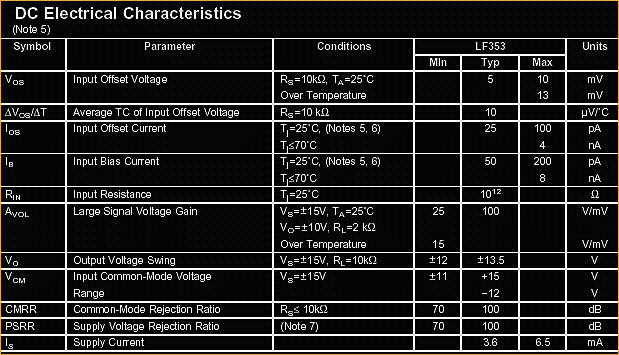• Input Offset Voltage:
This tells you how much the output voltage might differ from the expected value. For the regulator above, the output of the regulator might vary by the value given by this spec. If the voltage on the positive input is 10.35 volts, the output voltage could be 10.35 ±5mv for this IC.
• Delta Vos/Delta T:
This tells you how the offset voltage will be affected by temperature.
• Input Bias Current:
This is the amount of current the input circuit will need to properly operate. It is a very small value (generally a few pico amps).
• Input Resistance:
This is self explanatory. What you should notice is how high the resistance is (10,000,000,000,000 ohms). This is why op amps make such good buffers. It would have virtually no effect on the circuit to which it is connected. Few things are affected by a circuit with 10 trillion ohms of input resistance.
• Output Voltage Swing:
This tells you how far the voltage can swing with a given power supply voltage. It is rare that the op amp can swing to the full power supply voltage. Op amps that can swing to very near the power supply voltage are called 'Rail to Rail' op amps. They are generally much more expensive than op amps like the LF353. Most circuits in crossovers and equalizer that use op amps, have a power supply that delivers ±15 volts DC. This means that the output voltage could be as high as 13 volts peak (or 9.2 VRMS). Since you rarely need more than a few volts to drive an amplifier into clipping, having rail to rail op amps isn't necessary. Rail to Rail op amps would more likely be found in devices that only have a single ended regulated supply such as you'd find in a head unit.
• Common-Mode Rejection Ratio:
This tells you how far down a signal would be if it was driven equally into both inputs. If you had a 1 volt of desired signal (a 1khz sine wave perhaps) driven into one of the inputs and and a 1 volt signal (a 2khz sine wave) driven into BOTH inputs, at the op amp's output the 2khz signal would be a given number of dBs down from the level driven into the inputs. In this case, it's at least 70dB down. This number is important to know if you're designing a circuit with a balanced signal. If the balanced line were to pass through an electromagnetically noisy environment (where noise would be induced into both signal lines), this is an indication of how the noise would be cancelled out/removed from the desired signal output.
• Power Supply Rejection Ratio:
This expresses how much the noise on the power supply will be suppressed.

AC Characteristics:• Amp-Amp Coupling:
Virtually all amplifiers with multiple channels have crosstalk between channels. This is where one channel bleeds into the other channel. If one of the op amps in this package had an output voltage of 15 volts, the second op amp would have an output 120dB lower (even if the second op amp had no signal connected to it's input terminals).
• Slew Rate:
This spec tells you how fast the op amp can drive its output from one voltage to another. If it can transition at 13 volts per microsecond, it can (with a power supply voltage of ±15 volts) go from its full negative output voltage to its full positive output voltage in `2 microseconds. For audio, virtually all op amps have a sufficient slew rate to be able to reproduce the entire audio bandwidth.
• Gain Bandwidth Product:
This expresses the op amps ability to provide gain across its operable bandwidth. For this op amp (GBW = 4MHZ) it can be used with a 4 megahertz bandwidth at unity gain (gain = 1). At a gain of 2, it would only be good for a 2 MHZ bandwidth. At a gain of 10, it would only be useable from DC to 400khz. The higher the gain, the narrower bandwidth.

```

```